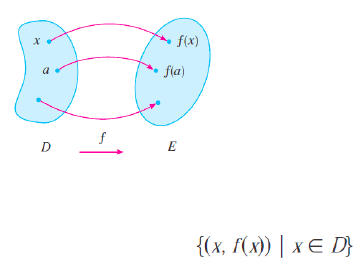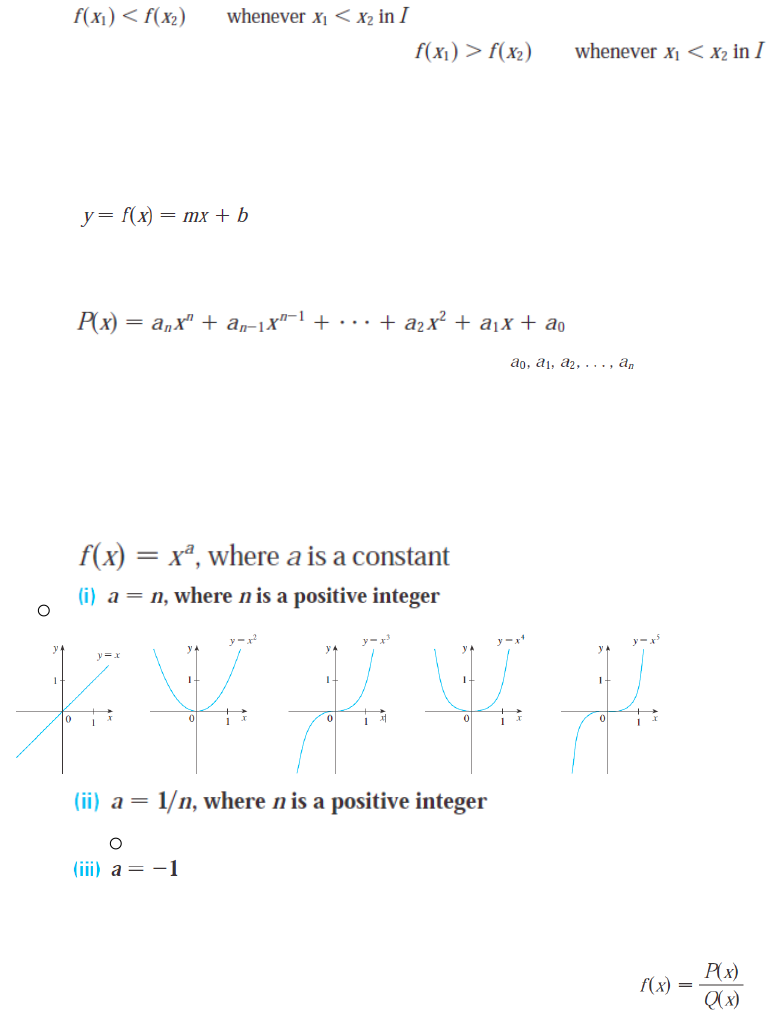Textbook Notes (270,000)
CA (160,000)
UTSG (10,000)
MAT (300)
all (10)
Study Guide

# [MAT133] - Final Exam Guide - Everything you need to know! (197 pages long)

Department
Mathematics
Course Code
MAT133Y1
Professor
all

This preview shows pages 1-3. to view the full 197 pages of the document.Only pages 1-3 are available for preview. Some parts have been intentionally blurred.Functions and Models
1.1 Four ways to represent a function
Representations of functions
oA function f is a rule that assigns to each element x in a set D
exactly one element, called f(x), in a set E.
oThe set D is called the domain of the function.
oThe number f(x) is the value of f at x and is read “f of x.”
oThe range of f is the set of all possible values of f(x) as x
varies throughout the domain.
oA symbol that represents an arbitrary number in the domain of
a function f is called an independent variable. A symbol that
represents a number in the range of f is called a dependent
variable.
oAnother way to picture a function is by an arrow diagram.
oThe most common method for visualizing a function is its
graph. If f is a function with domain D, then its graph is the set
of ordered pairs .
oThere are four possible ways to represent a function:
Verbally (by a description in words)
Numerically (by a table of values)
Visually (by a graph)
Algebraically (by an explicit formula)
The Vertical Line Test A curve in the xy-plane is the graph of a
function of x if and only if no vertical line intersects the curve more
than once.
Functions that are defined by different formulas in different parts of
their domains are called piecewise defined functions.
Symmetry:
oIf a function f satisfies f(-x)=f(x) for every number x in its
domain, then f is called an even function. The geometric
significance of an even function is that its graph is symmetric
with respect to the y-axis.
oIf f satisfies f(-x)=-f(x) for every number x in its domain, then is
called an odd function. The graph of an odd function is
symmetric about the origin.

Only pages 1-3 are available for preview. Some parts have been intentionally blurred.Increasing and decreasing functions:
oA function f is called increasing on an interval I if
oIt is called decreasing on I if
1.2 Mathematical Models: A catalog of essential functions
Linear Models
owhere m is the slope and b is the y-intercept.
Polynomials
oA function is called a polynomial if
where n is a
nonnegative integer and the numbers are
constants called the coefficients of the polynomial.
oThe degree of the polynomial is n.
oA polynomial of degree 2 is called a quadratic function.
oA polynomial of degree 3 is called a cubic function.
Power Functions
o
o
o
oIt’s called a root function.
o
oIt’s called a reciprocal function.
Rational Functions
oA rational function f is a ratio of two polynomials:
Algebraic Functions
oA function is called an algebraic function if it can be
constructed using algebraic operations (such as addition,
subtraction, multiplication, division, and taking roots) starting
with polynomials.
Trigonometric Functions
###### You're Reading a Preview

Unlock to view full version

Only pages 1-3 are available for preview. Some parts have been intentionally blurred.###### You're Reading a Preview

Unlock to view full version